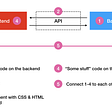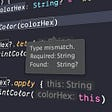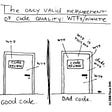# Problem

There are N houses for sale. The i-th house costs Ai dollars to buy. You have a budget of B dollars to spend.

What is the maximum number of houses you can buy?

# Input

The first line of the input gives the number of test cases, T. T test cases follow. Each test case begins with a single line containing the two integers N and B. The second line contains N integers. The i-th integer is Ai, the cost of the i-th house.

# Output

For each test case, output one line containing `Case #x: y`, where `x` is the test case number (starting from 1) and `y` is the maximum number of houses you can buy.

# Limits

Time limit: 15 seconds per test set.
Memory limit: 1GB.
1 ≤ T ≤ 100.
1 ≤ B ≤ 105.
1 ≤ Ai ≤ 1000, for all i.

1 ≤ N ≤ 100.

1 ≤ N ≤ 105.

# Sample

Input

`34 10020 90 40 904 5030 30 10 103 300999 999 999`

Output

`Case #1: 2Case #2: 3Case #3: 0`

Explanation

In Sample Case #1, you have a budget of 100 dollars. You can buy the 1st and 3rd houses for 20 + 40 = 60 dollars.
In Sample Case #2, you have a budget of 50 dollars. You can buy the 1st, 3rd and 4th houses for 30 + 10 + 10 = 50 dollars.
In Sample Case #3, you have a budget of 300 dollars. You cannot buy any houses (so the answer is 0).

Note: Unlike previous editions, in Kick Start 2020, all test sets are visible verdict test sets, meaning you receive instant feedback upon submission.

Code & Algorithm

`/* author : @akash kumar */#include<bits/stdc++.h>using namespace std;#define ll long long int#define mod 1000000007#define pb push_back#define ld long doublevoid solve(){  int n;      int bg;      cin>>n>>bg;      int a[n] ;      for(int i=0;i<n;++i)      {       cin>>a[i];      }      int ans=0;      int c=0;      for(int i=0;i<n;++i)      {       ans+=a[i];       if(ans<=bg)       {        c++;       }       else       {        break;       }      }      cout<<c; }int main(){    ios_base::sync_with_stdio(0);    cin.tie(0);    cout.tie(0);    int t;    cin>>t;    for(int i=1;i<=t;++i)    {       cout<<"Case #"<<i<<": ";       solve();       cout<<"\n";    } return 0;}`

We want to buy as many as possible houses. Intuitively, we can keep buying the cheapest house. The rationale is to save money at each step so we could buy more in the end. One way to implement this strategy is to sort all the houses by prices from low to high and then buy houses one by one until we run out of money.

The sorting part has O(N log N) time complexity and the processing part has O(N) time complexity. Using counting sort could reduce the sorting complexity to O(N) since the range of the prices is [1, 1000]. The overall time complexity is O(N).

Let’s prove the correctness of this greedy algorithm. Let the solution produced by the greedy algorithm be A = {a1, a2, …, ak} and an optimal solution O = {o1, o2, …, om}.

If O and A are the same, we are done with the proof. Let’s assume that there is at least one element oj in O that is not present in A. Because we always take the smallest element from the original set, we know that any element that is not in A is greater than or equal to any ai in A. We could replace oj with the absent element in A without worsening the solution, because there will always be element in A that is not in O. We then increased number of elements in common between A and O, hence we can repeat this operation only finite number of times. We could repeat this process until all the elements in O are elements in A. Therefore, A is as good as any optimal solution.

Thank You.

Akash Kumar

Software Engineer

--

--

--

Love podcasts or audiobooks? Learn on the go with our new app.

## Do you keep a tidy Git commit history?## Setting Up Git for Unity## alert(“My TIIDELab experience version” + “ 3.0 (Third Month)”)## “Static Data Member & Member Function in C++”## Lighthouse CI for Page Performance Testing and History Keeping## Testing modern web apps, Part 1: The big picture## The correct way to do null checks in Kotlin. FINALLY!!## Algorithm: Solve Sudoku## What is Reactive Programming? | Interview basics## Keeping it Clean — the Art of Clean Coding## Flatiron Phase 1 // Week 1